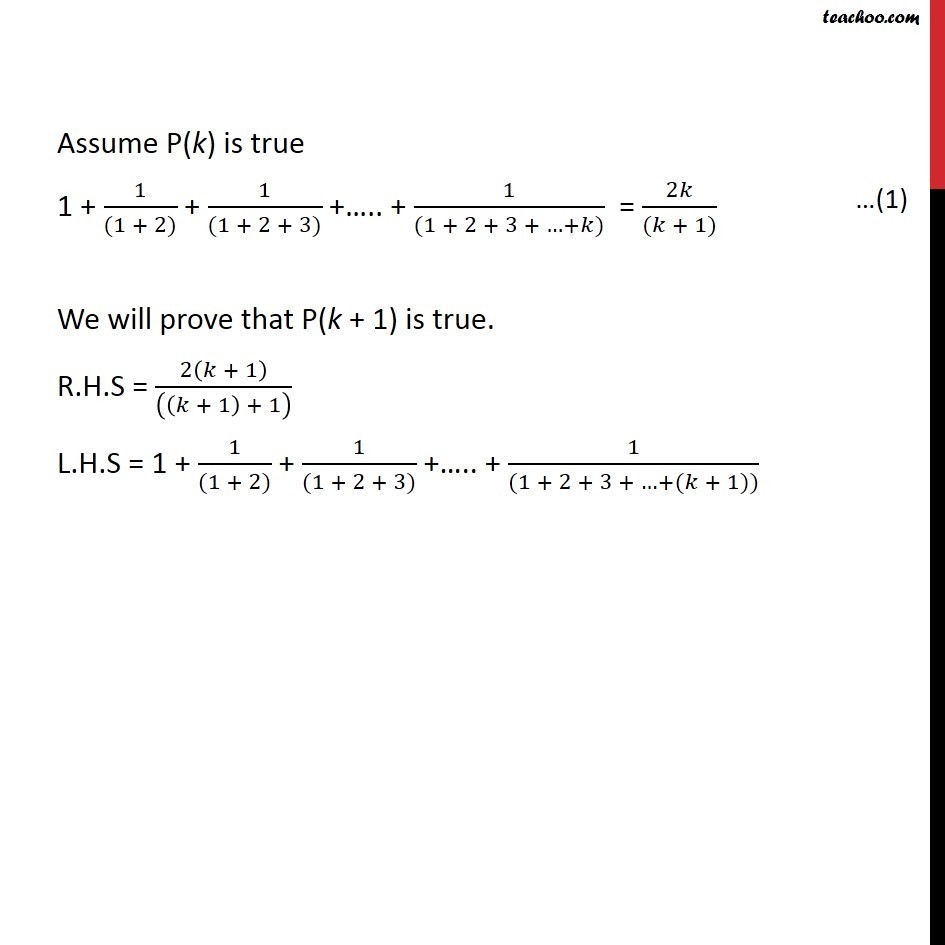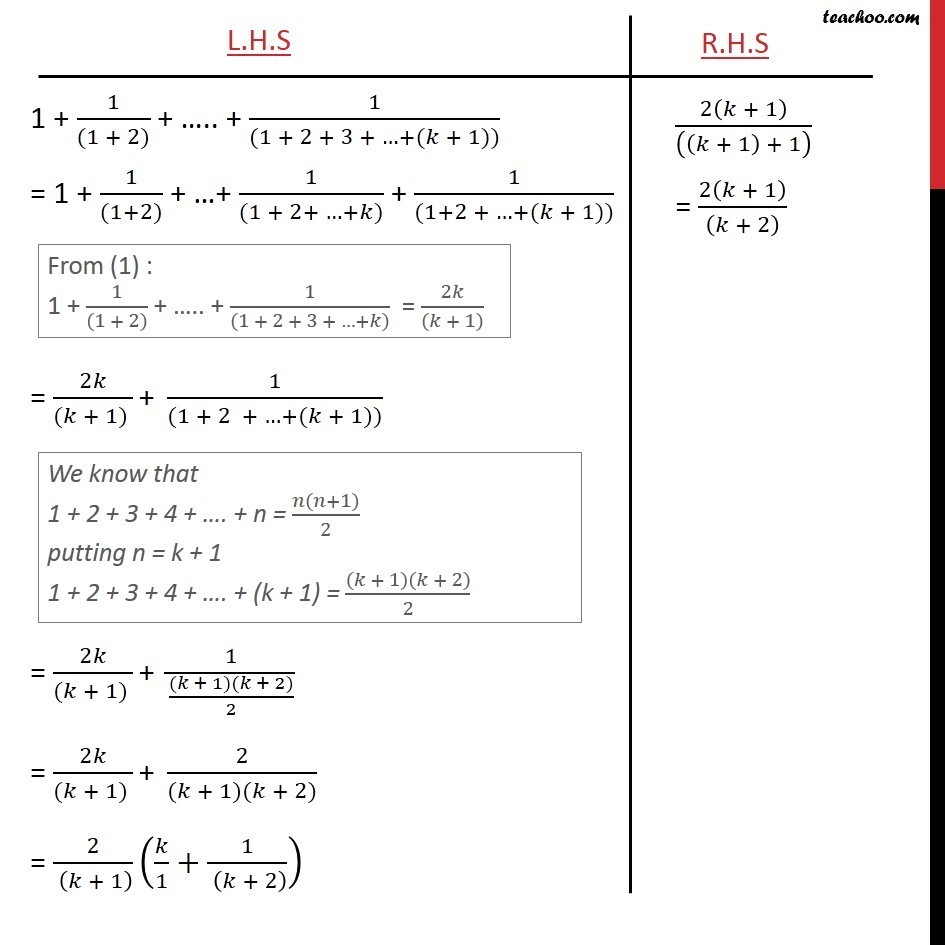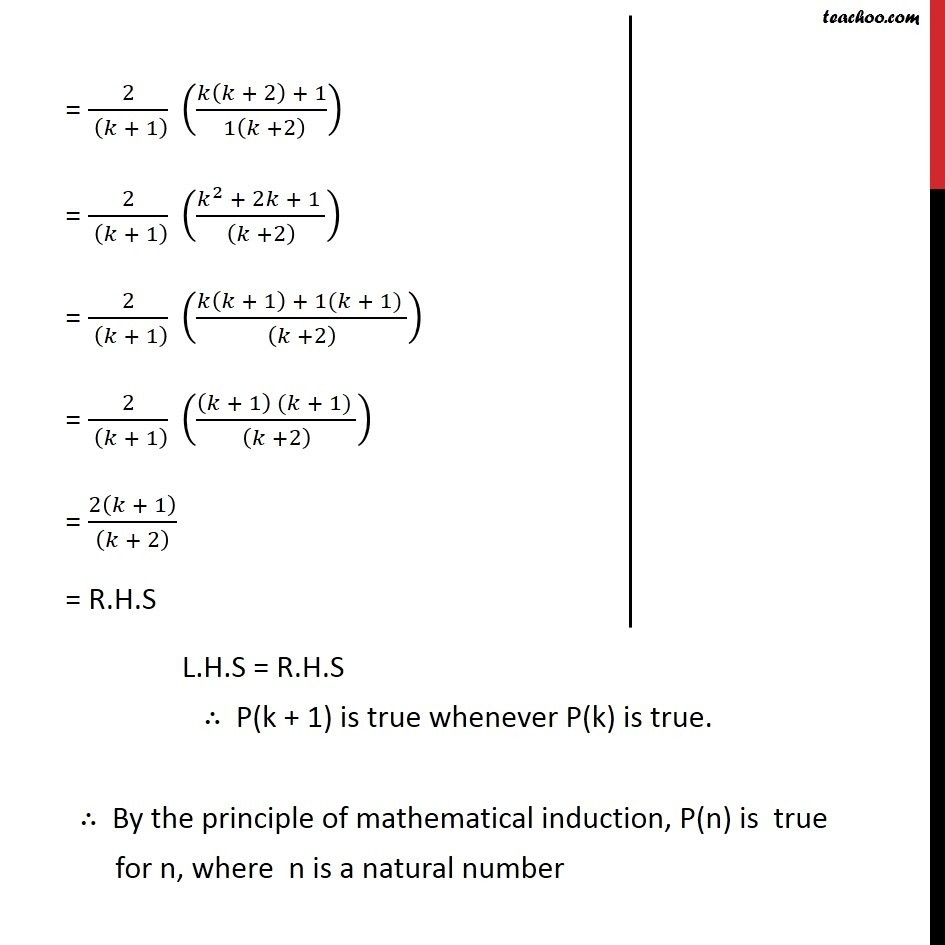Mathematical Induction - Questions and Solutions

Mathematical Induction
Serial order wiseLearn in your speed, with individual attention - Teachoo Maths 1-on-1 Class

### Transcript

Question3: Prove the following by using the principle of mathematical induction for all n N: 1 + 1/((1 + 2)) + 1/((1 + 2 + 3)) + .. + 1/((1 + 2 + 3 + . )) = 2 /(( + 1)) Let P (n) : 1 + 1/((1 + 2)) + 1/((1 + 2 + 3)) + .. + 1/((1 + 2 + 3 + . )) = 2 /(( + 1)) For n = 1, L.H.S = 1 R.H.S = 2(1)/(((1) +1)) = 2/((2)) = 1 Hence, L.H.S. = R.H.S , P(n) is true for n = 1 Assume P(k) is true 1 + 1/((1 + 2)) + 1/((1 + 2 + 3)) + .. + 1/((1 + 2 + 3 + + )) = 2 /(( + 1)) We will prove that P(k + 1) is true. R.H.S = 2( + 1)/((( + 1) + 1) ) L.H.S = 1 + 1/((1 + 2)) + 1/((1 + 2 + 3)) + .. + 1/((1 + 2 + 3 + +( + 1)))